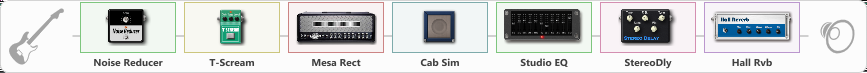Discussion in 'ToneLib-GFX presets' started by Roberto Ilo Horst, Jan 27, 2022.

1. ### Roberto Ilo HorstNew Member

Using a superstrat's bridge humbucker pickup..

Effects chain:Effect: "Noise Reducer" (Dynamics / Filter), active - "yes"
{
"Sens" = 60
"Mode" = Soft
}

Effect: "T-Scream" (Overdrive / Distortion), active - "yes"
{
"Drive" = 0
"Tone" = 94
"Level" = 92
}

Effect: "Mesa Rect" (Amp simulators), active - "yes"
{
"Gain" = 51
"Bass" = 43
"Middle" = 81
"Treble" = 58
"Presence" = 58
"Master" = 66
"Level (dB)" = 2
}

Effect: "Cab Sim" (Cabinets), active - "yes"
{
"Model" = 4x12" 1960 T75
"Level (dB)" = 3
}

Effect: "Studio EQ" (Dynamics / Filter), active - "yes"
{
"31 Hz" = -6
"62 Hz" = -1
"125 Hz" = 4
"250 Hz" = -3
"500 Hz" = -3
"1 kHz" = 10
"2 kHz" = 0
"4 kHz" = 2
"8 kHz" = -1
"16 kHz" = -4
"Level (dB)" = 0
}

Effect: "StereoDly" (Delay), active - "yes"
{
"Time" = 500
"Feedback" = 44
"Tone" = 76
"Sens" = 0
"Mix" = 61
}

Effect: "Hall Rvb" (Reverberation), active - "yes"
{
"Time" = 6.9
"PreDelay" = 32
"LoDamp" = 0
"HiDamp" = 2
"Mix" = 32
}

Note: You will need to download and install the ToneLib-GFX software to use the preset.